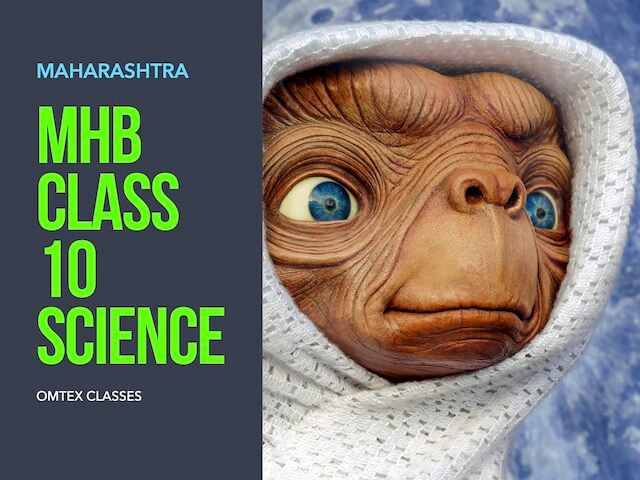### Question 4. Write the three laws given by Kepler. How did they help Newton to arrive at the inverse square law of gravity?

Question 4.

Write the three laws given by Kepler. How did they help Newton to arrive at the inverse square law of gravity?

Johannes Kepler studied about the planets motion and their positions and. He noticed that motion of the planets follows a certain law. He gave three laws describing the planetary motion. These are known as Kepler’s laws which are as follows:

Kepler’s First law: The orbit of planet is an ellipse with sun at one of the foci. The figure below illustrates the elliptical orbit of earth with sun at its focus.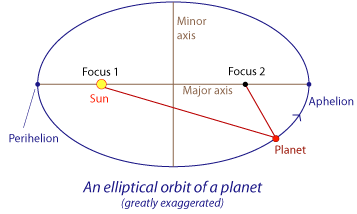Kepler’s Second law: The line joining the planet and the Sun sweeps equal areas in equal intervals of time.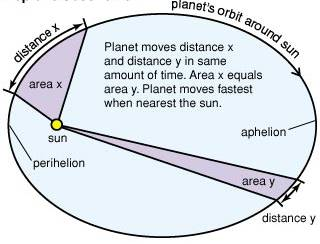In the above figure A1 = A2 according to law.

Kepler’s Third law: The Square of its period of revolution around the Sun is directly proportional to the cube of the mean distance of a planet from the Sun.

If ‘r’ is the average distance of the planet from the sun and ‘T’ is the period of revolution then according to third law;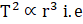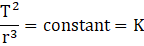Inverse Square law of gravity: We know that centripetal force F is given by ;

F =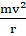Where v = velocity of the planet =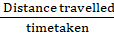Distance travelled in one revolution=2πr

Where r = radius of the orbit.

V =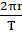F =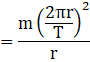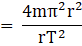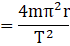According to Kepler’s third law;Hence

F =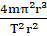(Multiplying and dividing by r2)

F =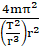F =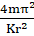(By Kepler’s third law).

But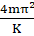is constant

Therefore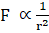And this is the newton’s inverse square law which states that the centripetal force acting on planet is inversely proportional to the square of the distance (‘r’) between the sun and the planet